# Modal parameter identification and finite element model updating of a long-span aqueduct structure based on ambient excitation

## Liguo Sun1, Yezhuang Xu2

1College of Mechanics and Materials, Hohai University, Nanjing, 211100, China

2Highway Development Center, Department of Transport of Jiangsu Province, Nanjing, 210001, China

1Corresponding author

Journal of Vibroengineering, Vol. 22, Issue 4, 2020, p. 896-908. https://doi.org/10.21595/jve.2020.21271
Received 7 October 2019; received in revised form 19 December 2019; accepted 6 January 2020; published 30 June 2020

Copyright © 2020 Liguo Sun, et al. This is an open access article distributed under the Creative Commons Attribution License, which permits unrestricted use, distribution, and reproduction in any medium, provided the original work is properly cited.
Views 134
Abstract.

In this paper, PSV-500 laser vibration detector and 941B vibration pick-up are used to measure the ambient vibration of an actual aqueduct in China, and the peak picking method is used to identify the modal parameters of the aqueduct. The finite element model of the aqueduct is established, and a model updating method based on multi-objective optimization algorithm is proposed. Based on the sensitivity analysis, the parameters to be updated are selected. The model is updated by the fast non dominated sorting genetic algorithm, and the Pareto optimal solution set of the multi-objective optimization problem is obtained. The comparison between the measured and calculated results shows that the results of static displacement and modal parameters are in good agreement with the measured values. The result of the research shows that the static and dynamic finite element model updating method based on multi-objective optimization can achieve satisfactory results for long-span aqueduct structure, and the updated finite element model can accurately and comprehensively simulate the actual structure.

Keywords: modal parameter identification, peak picking method, model updating, non-dominated sorting genetic algorithm.

#### 1. Introduction

According to different construction materials, in China, aqueducts can be divided into masonry aqueducts, concrete aqueducts and reinforced concrete aqueducts. Limited by the engineering technology and material applications during the construction period, in the past few decades, many masonry arch aqueducts had been built in China. Especially in the 1970s, due to the lack of cement and steel, grouted rubble, with these advantages of local materials and low construction cost, has been widely used. However, after a long-term operation, diseases such as aging and damage appear in aqueducts and thereby result in structural damage or performance degradation. Therefore, it is critical important to diagnose and evaluate the health status of aqueduct. For the aqueduct with a large structural damage and performance degradation, an effective reinforcement scheme need to be proposed to ensure the safety of aqueduct in the future operation . For aqueduct structures with structural damage and performance degradation, the existing nondestructive testing technology [2-7] is difficult to identify the exact shape, size and distribution of defects in the large massive structure. Therefore, it is difficult to accurately evaluate the damage and degradation degree of the structure. Based on relevant test data and effective advanced numerical methods, the inversion identification model of large-scale structural defects is studied [8-10], which can accurately and efficiently identify the location, size and type of defects, and provide a new method and idea for defect detection and safety identification of large-scale structures, as well as a reliable basis for structural reinforcement and repair. However, the premise of the above-mentioned work is to need a benchmark finite element model which can meet engineering accuracy requirements and reflect structural authenticity.

The available modal frequency, mode shape and damping modal parameters can be used to evaluate the safety status of the aqueduct structure [11-13]. However, due to the simplification error of boundary conditions, the mesh discretization error, and the material parameters error and so on, a gap may exist between the predicted structural response and the actual structural response. To improve the prediction accuracy, it is necessary to modify the finite element model. That is, on the premise of ensuring the accuracy of modal parameters and the clear physical meaning of updated parameters, the static and dynamic measured results can be used to modify the relevant parameters of finite element model, so that the computational results can be consistent with the measured values.

The updating of finite element model is essentially an optimization problem. The issue is to construct a reasonable objective function which contains the static and dynamic response information. Different combination of static and dynamic data can form different objective functions. Based on the measured static and dynamic data, many scholars have put forward many effective methods for the finite element model updating of various structures or indoor models [14-18]. But most of these methods use the linear weighted summation method to establish the optimization objective function, that is, each objective vector is given a weight, and each objective component is multiplied by its corresponding weight coefficient and then combined into a new objective function. Thus, it is transformed into a single objective optimization problem. The disadvantage of this method is that although the relative importance of each objective is taken into account in the weight assignment, the selection of weight coefficient is still subjective. In this paper, the static and dynamic finite element model updating based on multi-objective optimization is proposed and then the method is applied to the finite element model updating of masonry aqueduct.

#### 2.1. Arrangement of monitoring points

The studied aqueduct, located in Tiantai County, Zhejiang, is divided into 5 spans. Each span is 36 m long. The main arch ring is circular arc, and the center angle is 116°32'. The length of the inlet and outlet section is about 19 m, and the maximum height from the top of the trench to the riverbed is 21.5 m. The aqueduct body is 5.24 m wide and 3.5 m high, the sidewalks on both sides of the bank are 0.8 m wide, and the trapezoid cross section has a design flow of 12.2 m3/s. The main materials include: 50# masonry block stone groove body, 100# masonry strip stone arch ring, 50# masonry block stone arch abdomen, 80# masonry strip stone pier surface, 75# masonry block stone pier body, 150# concrete pier cap, and 200# sidewalk frame on the top of the groove on the right bank. The pier is poured by underwater concrete with pipe piles of grab hammer. The depth of the column is 12 m, and the bottom of the pile is deep into the bedrock. There are 4 pile foundations for each pier foundation. Fig. 1 shows the profile of the aqueduct.

Fig. 1. An actual aqueduct in China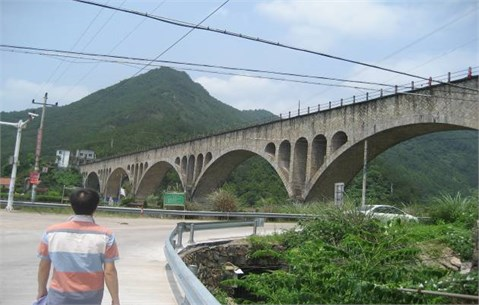Fig. 2 shows eight horizontal vibration measuring points arranged on the top of the left half of aqueduct according to the general vibration law of aqueduct structure. The sensor used for base point vibration test is the 941B vibration pickup produced by the Institute of engineering mechanics, China Earthquake Administration. The other seven vibration measuring points are measured by scanning with PSV-500 laser vibrometer produced by German Polytec company. This test method reduces the wiring and improves the test efficiency and accuracy. The vibration signal is connected to DH5920 dynamic data acquisition system through 941 special amplifier for acquisition. Fig. 3 shows the test site.

Fig. 2. Arrangement of aqueduct vibration measuring points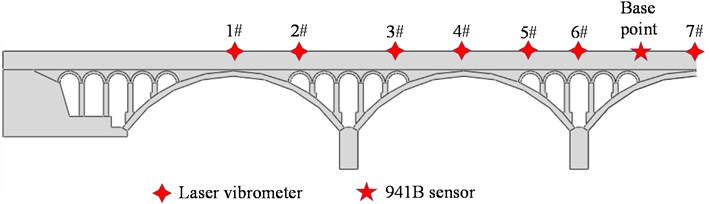Fig. 3. Laser scanning vibration measurement site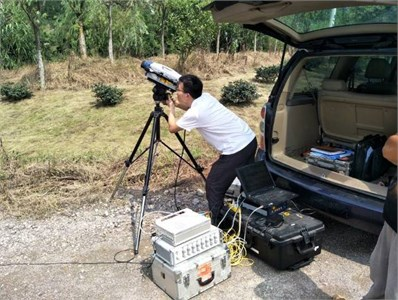#### 2.2. Modal test results

Since the input of aqueduct structure is inconvenient to measure in practical application, the identification of natural frequency is often based on the auto power spectrum of structural response. However, due to the interference of measurement noise and excitation spectrum, the peak value of structure auto power spectrum is not necessarily the modal frequency. According to the following principles, the structural modal frequency can be determined by the difference of the structural response frequency characteristics: (1) the peak value of the auto power spectrum of each measuring point of the structural response is located at the same frequency; (2) the coherence function between the measuring points at the modal frequency is larger; (3) each measuring point has the characteristics of approximately the same phase or opposite phase at the modal frequency.

In this test, the vibration displacement signal of aqueduct is directly obtained by integration. Each working condition is tested for 30 minutes, and the frequency is 50 Hz. According to the displacement time history of each measuring point obtained from the ambient vibration test, the modal parameters of aqueduct structure can be identified by using the auto cross spectrum density method. In the case of unknown excitation force, based on a series of idealized assumptions of the input signal and the structure, the modal parameters of the system are identified by using five graphs of auto-power spectrum output from the response point of the structure and the amplitude, phase, coherence function and transmissibility of the cross-power spectrum output from the reference point. Power spectrum and cross power spectrum are adopted instead of frequency response function. The characteristic frequency is determined by the peak value on the power spectral density curve; the damping ratio is obtained by traditional half power bandwidth method; the vibration mode component is determined by the value of transmission rate at the characteristic frequency, and the direction is determined by the cross power spectral phase or the real part symbol of the transmission rate. This method can identify the modal parameters quickly, and is suitable for the preliminary and rapid determination of the structural modal frequency in engineering monitoring site.

According to the displacement time history obtained from the ambient vibration test, the amplitude, phase and coherence function of the auto cross power spectrum of the response point and the reference point can be calculated, and the spectral line is illustrated in Fig. 4. The average periodogram method is used to calculate the power spectral density function, and the data point of fast Fourier transform is 1024. Hamming window is added, the window length is 1024, and the data overlap rate is 50 %.

Fig. 4. Amplitude, phase and coherence function curve of auto and cross power spectrum of each measuring point and base point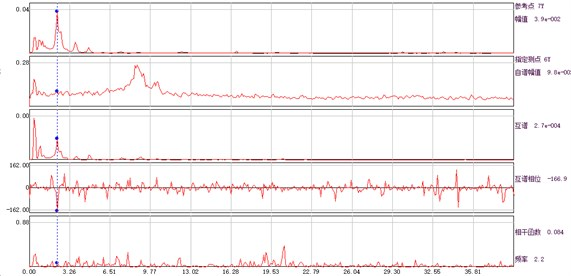a) Measuring point 1# and base point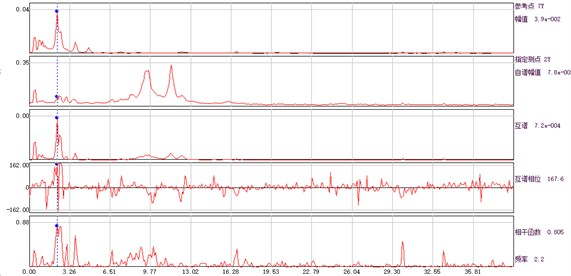b) Measuring point 2# and base point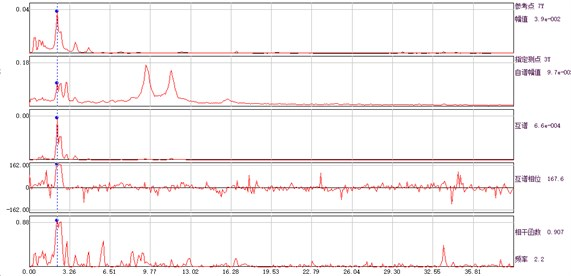c) Measuring point 3# and base point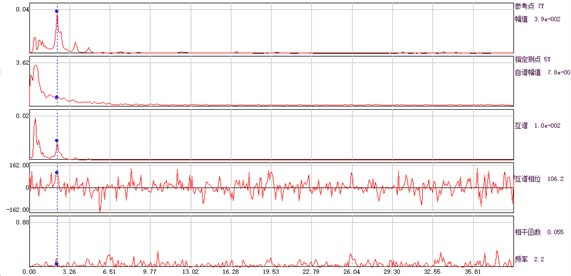d) Measuring point 4# and base point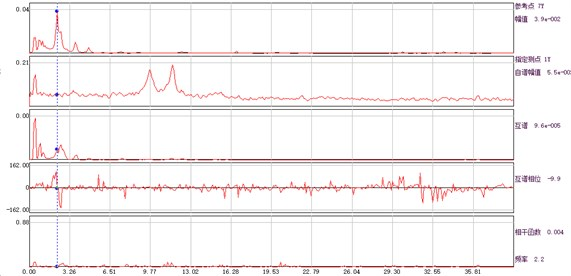e) Measuring point 5# and base point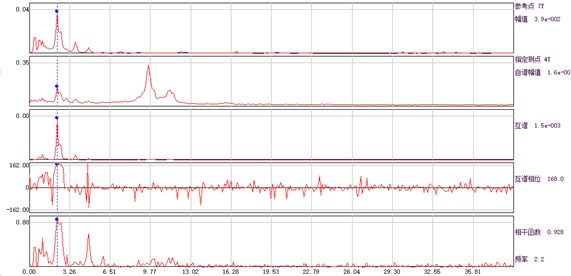f) Measuring point 6# and base point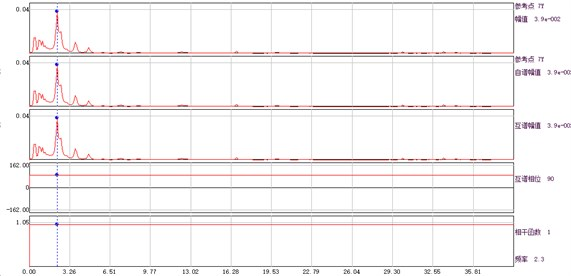g) Measuring point 7# and base point

The identified first three frequencies and modes by using the auto and cross power spectrum method are shown in Table 1. For the convenience of finite element model updating, the standard vectors are normalized in this paper.

Table 1. Frequency and mode vector of measuring point

 Order Frequency / Hz Normalized mode vector of measuring point 1 2.25 ${\left[0.20,0.35,0.60,0.76,0.85,0.86,1.00\right]}^{T}$ 2 2.54 ${\left[-0.47,-0.69,-0.95,-1.00,-0.77,-0.55,-0.10\right]}^{T}$ 3 2.93 ${\left[-0.80,-0.94,-0.76,-0.40,0.12,0.51,1.00\right]}^{T}$

#### 3.1. Arrangement of settlement monitoring points

The settlement of aqueduct is measured by static leveling method, and the monitoring points are arranged on the middle line of pedestrian passage on both sides of aqueduct. The monitoring point HX Base-1 is located at the mountain at the downstream end of aqueduct, and the other measuring points are located at the 1/4 span, 1/2 span, 3/4 span and two ends of each span. The arrangement of 46 monitoring points is showed in Fig. 5.

Fig. 5. Arrangement of monitoring points for settlement observation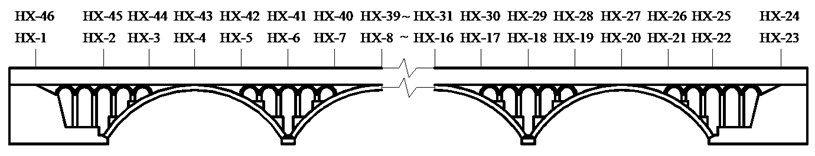a) Vertical section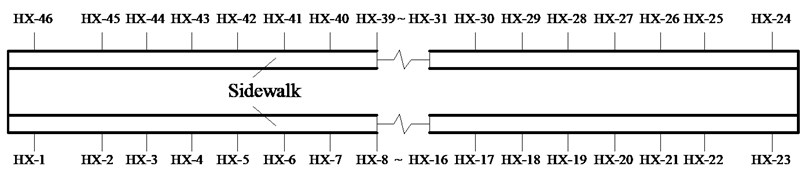b) Plan section

#### 3.2. Test methods and results

One vertical displacement reference point (HX Base-1) is set at both ends of the suspension span far away from the aqueduct, where the geological conditions are favorable and stable. HX base-1 and 45 monitoring points form a vertical displacement monitoring network. Observation is carried out according to first-class leveling requirements, and precision index is carried out according to second-class leveling standards. In the case the aqueduct stops flowing water and there exists no water, a closed line measurement can be made 12 hours later and recorded as the original data. After the aqueduct water flow reaches the stable value, it waits for 12 hours to measure and record the closed line, and the settlement data is obtained through data analysis based on the original record.

Before and after the aqueduct is flooded, the height difference of each measuring point relative to the base point (HQ Base-1) is measured respectively, and the settlement deformation data of aqueduct is obtained. Fig. 6 shows the vertical displacement of each measurement point.

Fig. 6. Measured vertical displacements of the aqueduct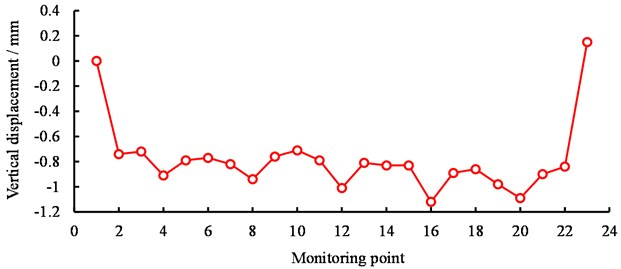a) Monitoring point on the left sidewalk (HX1~HX23)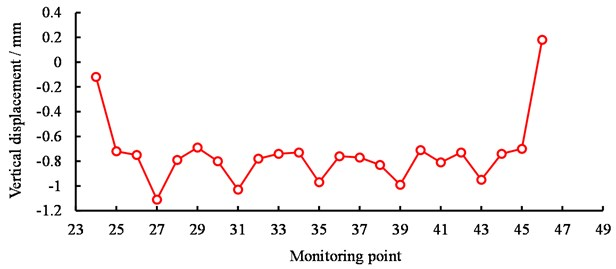b) Monitoring point on the right sidewalk (HX24~HX46)

#### 4.1. Objective function

Based on the measured vibration frequency and the measured vertical displacement, a multi-objective function is constructed. The objective function can be expressed as:

(1)
$\mathrm{m}\mathrm{i}\mathrm{n}\left\{{F}_{1},{F}_{2}\right\}=\left\{\sum _{i=1}^{n}{\left(\frac{{f}_{ai}-{f}_{ti}}{{f}_{ti}}\right)}^{2},\sum _{j=1}^{m}{\left(\frac{{U}_{aj}-{U}_{tj}}{{U}_{tj}}\right)}^{2}\right\},$

where, ${f}_{ai}$ and ${f}_{ti}$ are the calculated and measured $i$th order frequency of the aqueduct with empty resevior, respectively; ${U}_{aj}$ and ${U}_{tj}$ are the calculated and measured vertical displacement at the $j$th point of the aqueduct with full resevior; $n$ is the order of measured frequency; and $m$ is the number of settlement monitering point.

Since the calculated mode order of the finite element model does not necessarily coincide with the measured mode order, to ensure the mode matching between the calculated and the measured mode, the Modal Assurance Criterion (MAC)  is adopted to match the mode. The MAC can be expressed as follows:

(2)
$MAC=\frac{{\left|{\mathbf{\varphi }}_{a}^{T}{\mathbf{\varphi }}_{b}\right|}^{2}}{\left({\mathbf{\varphi }}_{a}^{T}{\mathbf{\varphi }}_{a}\right)\left({\mathbf{\varphi }}_{b}^{T}{\mathbf{\varphi }}_{b}\right)},$

where, ${\mathbf{\varphi }}_{a}$, ${\mathbf{\varphi }}_{b}$ are the calculated and measured mode vectors, respectively. MAC varies from 0 to 1, and the closer the MAC is to 1, the better the correlation is.

#### 4.2. Model updating scheme

Based on the measured dynamic parameters of aqueduct and the settlement under the design water level, the non dominated sorting genetic algorithm  is used to optimize the multi-objective function and seek a non inferior solution. The specific implementation of model updating is to combine the optimization algorithm with the finite element analysis to realize the optimization solution. Fig. 7 shows the flow chart of the model updating scheme.

The population size of the multi-objective optimization algorithm is 60, the maximum number of evolution is 80, the crossover probability is 0.9, and the mutation probability is 0.1.

Fig. 7. Flow chart of finite element model updating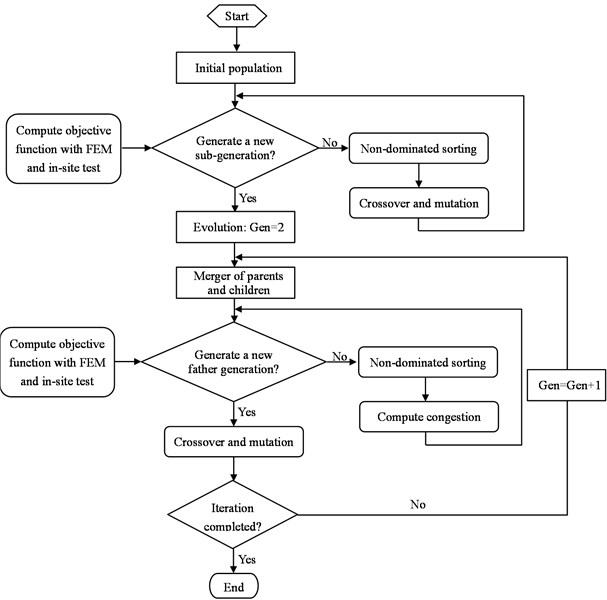#### 5.1. Finite element model

Fig. 8 shows the finite element mesh of the aqueduct and its associated auxiliary components including top water channel and lower support arch bridge. The whole structures were discretized into 8-node hexahedron isoparametric elements. The finite element model contained 20698-node and 13905-element.

Fig. 8. Finite element mesh#### 5.2.1. Updated parameter analysis

The parameter selection of model updating cover all possible error parameters, including the geometric parameters, material characteristic parameters and boundary conditions of the structure. According to the actual structure, the model is built in a scale of 1:1. The geometric parameters of the structure are not adjusted in the process of model updating. The masonry material for aqueduct construction, due to the incomplete construction technology in the 1970s and decades of use, has a large discrete type of strength and density. It is assumed that its adjustment range is ±10 % of the specified value. The measured settlement results with full resevior show that the settlement of the five arches of the aqueduct is not uniform. To make the updated model reflecting this characteristic, the arch ring parameters of the five arches are defined separately, but the initial values are the same. The initial material properties of finite element method is shown in Table 2.

In the process of updating, different parameters have different sensitivity to structural characteristic information. The following equation is used to calculate the relative sensitivity:

(3)
${S}_{ij}=\frac{\left[{f}_{i}\left({p}_{j}+∆{p}_{j}\right)-{f}_{i}\left({p}_{j}\right)\right]/{f}_{i}\left({p}_{j}\right)}{∆{p}_{j}/{p}_{j}},$

where, $∆{p}_{j}={p}_{j}/10$; ${p}_{j}$ is the median parameter; ${S}_{ij}$ represents the sensitivity of the relevant updating parameters of each index. In this paper, the structural response quantity $f$ is the characteristic quantity, i.e. modal frequency, mode shape and displacement and their combination. Table 3 shows the sensitivity of each parameter to the first three natural frequencies of the structure. According to the results of sensitivity analysis, it can be concluded that the density of the aqueduct body and the rigidity of the middle pier are most sensitive to the first three natural frequencies. After eliminating the parameters with less sensitivity, seven parameters, i.e. aqueduct body stiffness and density, large arch ring stiffness and density, small arch ring stiffness and density, and middle pier stiffness, are the selected parameters to be updated.

Table 2. Initial material properties

 Location Elastic modulus / GPa Density / (t/m3) Aqueduct body 7.2-8.8 1.62-1.98 Arch soffit 7.2-8.8 1.62-1.98 Large arch ring 13.5-16.5 2.25-2.75 Small arch ring 13.5-16.5 1.89-2.31 End pier 8.1-9.9 2.07-2.53 Middle pier 8.1-9.9 2.07-2.53

Table 3. Sensitivity of each parameter to the first three frequencies of the structure

 Location Elastic modulus Density 1st order 2nd order 3rd order 1st order 2nd order 3rd order Aqueduct body 0.02 0.06 0.12 –0.31 –0.31 –0.31 Arch soffit 0.00 0.01 0.01 0.00 0.00 0.00 Large arch ring 0.07 0.07 0.07 –0.11 –0.11 –0.11 Small arch ring 0.04 0.04 0.04 –0.06 –0.06 –0.06 End pier 0.00 0.00 0.01 0.00 0.00 0.00 Middle pier 0.35 0.30 0.22 0.00 0.00 0.00

#### 5.2.2. Model updating results

Fig. 9 illustrates the relative correction of updated structural parameters. It can be seen that the rigidity and density of aqueduct body and the rigidity of the small arch ring change by more than 8%, while the other parameters change relatively little. Table 4 shows the static displacement and measured values of each span endpoint and mid-span point before and after the model updating. Measured settlement of each measurement point after the model updating is more consistent with the calculated settlement. The measured and calculated maximum displacements occurred in the middle of the fourth span (HX-16 measuring point). Before the model updating, the difference between the calculated and measured deflections is 17.0 %, and after the updating, the difference is reduced to 0.9 %; the maximum error between the calculated and measured deflections of the initial model occur at the top point of the fifth span bearing (HX-22 measuring point), which decrease from 81.8 % before the updating to 10.4 %. The dynamic characteristics and measured natural frequency before and after the model updating are shown in Table 5.

Fig. 9. Relative correction of structural updating parameters / %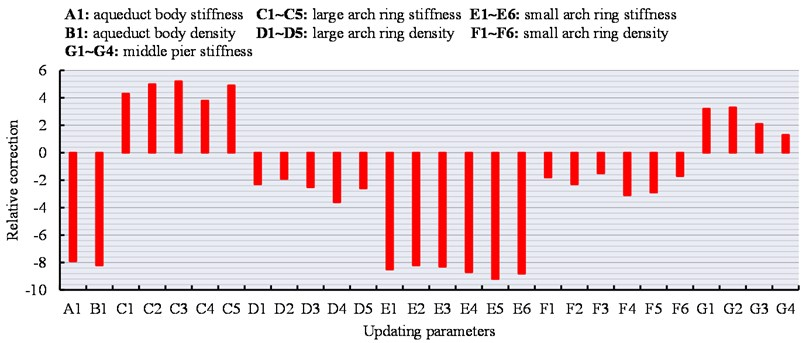Table 4. Comparison of settlement under design water level before and after model updating

 Measuring points Measured value/mm Calculated value Relative error/% Before updating After updating Before updating After updating HX-2 –0.73 –0.18 –0.71 75.3 2.7 HX-4 –1.01 –0.94 –1.02 6.9 1.0 HX-6 –0.73 –0.17 –0.69 76.7 5.5 HX-8 –0.99 –1.04 –1.05 5.1 6.1 HX-10 –0.73 –0.17 –0.70 76.7 4.1 HX-12 –0.99 –0.96 –1.00 3.0 1.0 HX-14 –0.80 –0.24 –0.75 70.0 6.3 HX-16 –1.06 –0.88 –1.05 17.0 0.9 HX-18 –0.84 –0.24 –0.80 71.4 4.8 HX-20 –1.02 –0.85 –1.04 16.7 2.0 HX-22 –0.77 –0.14 –0.69 81.8 10.4

Table 5. Comparison of dynamic characteristics before and after model updating

 Order Frequency MAC Measured / Hz Calculated value / Hz Relative error / % Before updating After updating Before updating After updating Before updating After updating 1 2.25 2.25 2.25 0 0 0.75 0.83 2 2.54 2.43 2.54 4.3 0 0.72 0.83 3 2.93 2.83 2.92 3.4 0.3 0.72 0.82

It can be concluded that after the updating, the deviation between the calculated natural frequency and the measured value of each order is obviously reduced, and the degree of coincidence is higher. The MAC value of each order is also improved and the updated MAC is greater than 0.80. The first three modes of aqueduct after updating are shown in Fig. 10. In summary, the static and dynamic finite element model updating based on multi-objective optimization can achieve better results when applied to the masonry aqueduct structure.

Fig. 10. First three modes of aqueduct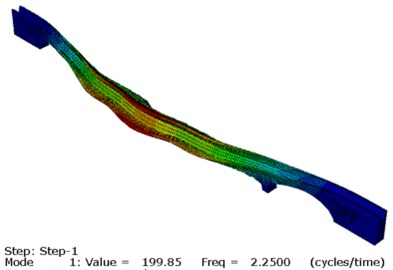a) The 1st mode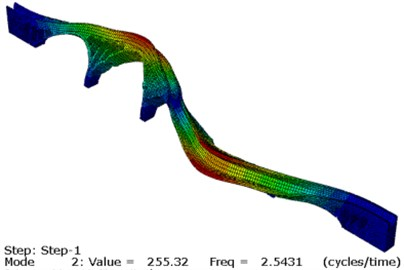b) The 2nd mode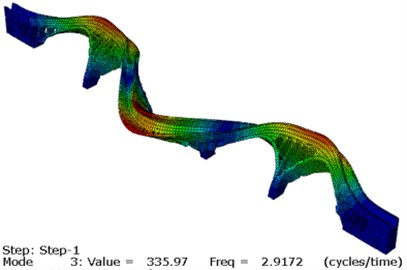c) The 3rd mode

#### 6. Conclusions

The static and dynamic finite element model updating method based on multi-objective optimization is proposed. By applying this method to the finite element model updating of a aqueduct, the following conclusions can be drawn:

1) The objective function is constructed based on the static and dynamic measured data. The updated results can reflect the static and dynamic characteristics of the masonry aqueduct more comprehensively, which are more reliable than those obtained by static or dynamic test response simply.

2) The calculated values of the first and second order frequencies are consistent with the measured values, and the calculated values of the third order frequencies slightly differ from the measured values by 0.3 %, indicating that the updated aqueduct model is closer to the actual values.

3) The fast non dominated sorting genetic algorithm is used to modify the finite element model of the aqueduct, and the Pareto non inferior solution set with uniform distribution is obtained. On this basis, the coordinated optimal solution of Pareto solution set is obtained by using the maximum bending angle method.

4) When the combination of multiple sub-objective functions is transformed into single objective functions in the existing joint static and dynamic model updating method, the subjective influence of artificial determination of weight coefficient on the model updating is inevitable. The static and dynamic finite element model updating method based on multi-objective optimization can not only avoid this kind of influence, but also achieves an ideal effect.

#### Acknowledgements

This work is supported by the Transportation Science Research Project of Jiangsu Province (No. 2019Z02), and the Fundamental Research Funds for the Central Universities (No. 2018B44514).

1. Gui J. P., Jiang S. H., Zhong H. H. Causes of dangerous situations for the Paizi River Aqueduct. Advances in Science and Technology of Water Resources, Vol. 28, Issue 3, 2008, p. 58-61, (in Chinese). [Search CrossRef]
2. Guo X. W., Vavilov V. Crack detection in aluminum parts by using ultrasound-excited infrared thermography. Infrared Physics and Technology, Vol. 61, 2013, p. 149-156. [Publisher]
3. Park H., Choi M., Park J., et al. A study on detection of micro-cracks in the dissimilar metal weld through ultrasound infrared thermography. Infrared Physics and Technology, Vol. 62, 2014, p. 124-131. [Publisher]
4. Rodriguez Martin M., Laguela S., Gonzalez Aguilera D., et al. Cooling analysis of welded materials for crack detection using infrared thermography. Infrared Physics and Technology, Vol. 67, 2014, p. 547-554. [Publisher]
5. Bigus G. A., Travkin A. A. An evaluation of the flaw-detection characteristics for the detection of fatigue cracks by the acoustic-emission method in samples made of steel 20 that have a cast structure. Russian Journal of Nondestructive Testing, Vol. 51, Issue 1, 2015, p. 32-38. [Publisher]
6. Carpinteri A., Lacidogna G., Accornero F., et al. Influence of damage in the acoustic emission parameters. Cement and Concrete Composites, Vol. 44, 2013, p. 9-16. [Publisher]
7. Boreiko D. A., Bykov I. Y., Smirnov A. L. The sensitivity of the acoustic-emission method during the detection of flaws in pipes. Russian Journal of Nondestructive Testing, Vol. 51, Issue 8, 2015, p. 476-485. [Publisher]
8. Deb K., Pratap A., Agarwal S., et al. A fast and elitist multiobjective genetic algorithm: NSGA-II. IEEE Transactions on Evolutionary Computation, Vol. 6, Issue 2, 2002, p. 182-197. [Publisher]
9. Zhao W., Du C., Jiang S. An adaptive multiscale approach for identifying multiple flaws based on XFEM and a discrete artificial fish swarm algorithm. Computer Methods in Applied Mechanics and Engineering, Vol. 339, 2018, p. 341-357. [Publisher]
10. Jiang S. Y., Zhao L. X., Du C. B. Identification of multiple flaws in structures based on frequency and modal assurance criteria. Chinese Journal of Theoretical and Applied Mechanics, Vol. 51, Issue 4, 2019, p. 1091-1100, (in Chinese). [Search CrossRef]
11. Gu P. Y., Wang L. L., Deng C., et al. Review of typical failure characteristics of aqueduct structures in China. Advances in Science and Technology of Water Resources, Vol. 37, Issue 5, 2017, p. 1-8, (in Chinese). [Search CrossRef]
12. Gu P. Y., Deng C., Wang L. L., et al. Modal test analysis of an aqueduct bent structure under artificial excitation. Journal of Vibration and Shock, Vol. 38, Issue 7, 2019, p. 146-154, (in Chinese). [Search CrossRef]
13. Gu P. Y., Liu D. M., Deng C. Modal test of aqueduct bent structures based on ambient excitation. Advances in Science and Technology of Water Resources, Vol. 39, Issue 4, 2019, p. 56-63, (in Chinese). [Search CrossRef]
14. Sanayei M., Khaloo A., Gul M., et al. CatbasAutomated finite element model updating of a scale bridge model using. Engineering Structures, Vol. 102, 2015, p. 66-79. [Publisher]
15. Wang H., Li A. Q., Li J. Progressive finite element model calibration of a long-span suspension bridge based on ambient vibration and static measurements. Engineering Structures, Vol. 32, Issue 9, 2010, p. 2546-2556. [Publisher]
16. Mosavi A. A., Sedarat H., O’connor S. M., et al. Calibrating a high-fidelity finite element model of a highway bridge using a multi-variable sensitivity-based optimisation approach. Structure and Infrastructure Engineering, Vol. 10, Issue 5, 2014, p. 627-642. [Publisher]
17. Brownjohn J. M. W., Moyo P., Omenzetter P., et al. Assessment of highway bridge upgrading by dynamic testing and finite-element model updating. Journal of Bridge Engineering, Vol. 8, Issue 3, 2003, p. 162-172. [Publisher]
18. Jaishi B., Ren W. X. Finite element model updating based on eigenvalue and strain energy residuals using multiobjective optimisation technique. Mechanical System and Signal Processing, Vol. 21, Issue 5, 2007, p. 2295-2317. [Publisher]
19. Berman A., Nagy E. J. Improvement of a large analytical model using test data. AIAA Journal, Vol. 21, Issue 8, 1983, p. 1168-1173. [Publisher]
20. Fox R. L., Kapoor M. P. Rates of eigenvalues and eigenvectors. AIAA Journal, Vol. 6, Issue 12, 1968, p. 2426-2429. [Publisher]

#### Cited By

 Nonlinear Engineering Wei Guo, Saeed Rubaiee, Anas Ahmed, Asem Majed Othman, Atul Khosla 2022 Structures Suzana Ereiz, Ivan Duvnjak, Javier Fernando Jiménez-Alonso 2022 Journal of Electrical Engineering & Technology HaoXin Zheng, MingHui Huang, LiHua Zhan, YanMei Zhu, PeiYao Liu 2021 Journal of Vibroengineering Haoxin Zheng, Hongbin Liu, Peiyao Liu 2021